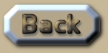Mass of the Universe

 Mass of the Universe Previously we calculated that the volume of the Universe is 1.1x10^70 km^3. And the density was seen to be 2.7x10^-18 kg/km^3. So the mass must be: 3x10^52 kg. .
 The reason why Sum Theory differs from all the other theories is that here Mass, Density, Volume, Radius, and the Shape of the Universe all add up precisely. If I had found another theory which had done this completely, I would have had nothing to say on the matter. The key features here are that the shape of the Universe does not seem to have been adequately appreciated to be a 3d-hyper-surface of a 4d-hypersphere. And also the straight-line radius is not the same as the curved distance light has traveled to reach us from the origin. (See previous section). .This is an extract summary of Chapter XXX of the book: Flight Light and Spin